##### Beginning Programming with Python For Dummies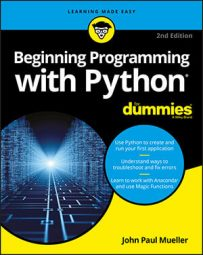A stack is a handy programming structure because you can use it to save a Python application execution environment (the state of variables and other attributes of the application environment at any given time) or as a means of determining an order of execution. Unfortunately, Python doesn’t provide a stack as a collection. However, it does provide lists, and you can use a `list` as a perfectly acceptable stack. The following steps help you create an example of using a `list` as a stack.
1. Type the following code into the notebook — pressing Enter after each line: `MyStack = []` `StackSize = 3` `def DisplayStack():` `print("Stack currently contains:")` `for Item in MyStack:` `print(Item)` `def Push(Value):` `if len(MyStack) < StackSize:``MyStack.append(Value)` `else:` `print("Stack is full!")` `def Pop():` `if len(MyStack) > 0:` `MyStack.pop()` `else:` `print("Stack is empty.")` `Push(1)` `Push(2)` `Push(3)` `DisplayStack()` `input("Press any key when ready...")```` Push(4)``` `DisplayStack()` `input("Press any key when ready...")` `Pop()` `DisplayStack()` `input("Press any key when ready...")` `Pop()` `Pop()` `Pop()` `DisplayStack()`

In this example, the application creates a `list` and a variable to determine the maximum stack size. Stacks normally have a specific size range. This is admittedly a really small stack, but it serves well for the example’s needs.

Stacks work by pushing a value onto the top of the stack and popping values back off the top of the stack. The `Push()` and `Pop()` functions perform these two tasks. The code adds `DisplayStack()` to make it easier to see the stack content as needed.

The remaining code exercises the stack (demonstrates its functionality) by pushing values onto it and then removing them. Four main exercise sections test stack functionality.

2. Click Run Cell.

Python fills the stack with information and then displays it onscreen. In this case, 3 is at the top of the stack because it’s the last value added.

Depending on the IDE you use, the `Press any key when ready` message can appear at the top or the bottom of the output area. In the case of Notebook, the message and associated entry field appear at the top after the first query. The message will move to the bottom during the second and subsequent queries.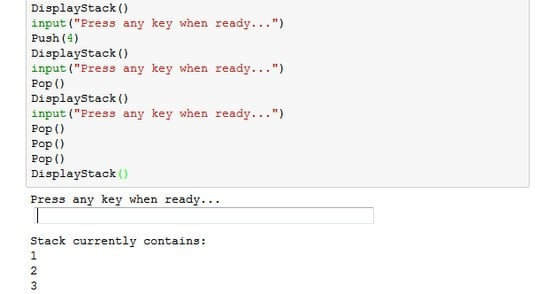A stack pushes values one on top of the other.
3. Press Enter.

The application attempts to push another value onto the stack. However, the stack is full, so the task fails.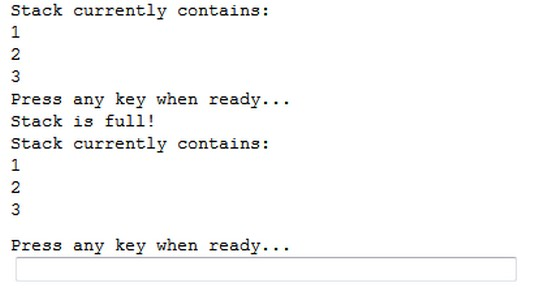When the stack is full, it can’t accept any more values.
4. Press Enter.

The application pops a value from the top of the stack. Remember that 3 is the top of the stack, so that's the value that is missing.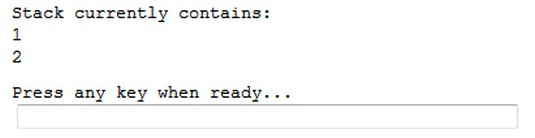Popping a value means removing it from the top of the stack.
5. Press Enter.

The application tries to pop more values from the stack than it contains, resulting in an error. Any stack implementation that you create must be able to detect both overflows (too many entries) and underflows (too few entries).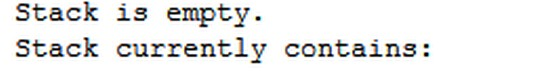Make sure that your stack implementation detects overflows and underflows.